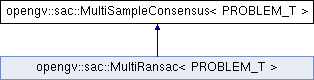OpenGV A library for solving calibrated central and non-central geometric vision problemsopengv::sac::MultiSampleConsensus< PROBLEM_T > Class Template Referenceabstract

`#include <MultiSampleConsensus.hpp>`

Inheritance diagram for opengv::sac::MultiSampleConsensus< PROBLEM_T >:## Public Types

typedef PROBLEM_T problem_t

typedef problem_t::model_t model_t

## Public Member Functions

MultiSampleConsensus (int maxIterations=1000, double threshold=1.0, double probability=0.99)
Constructor. More...

virtual ~MultiSampleConsensus ()
Destructor.

virtual bool computeModel (int debug_verbosity_level=0)=0
Fit the model to the data. More...

## Public Attributes

int max_iterations_

int iterations_

double threshold_

double probability_

model_t model_coefficients_

std::vector< std::vector< int > > model_

std::vector< std::vector< int > > inliers_

std::shared_ptr< PROBLEM_T > sac_model_

## Detailed Description

### template<typename PROBLEM_T> class opengv::sac::MultiSampleConsensus< PROBLEM_T >

Super-class for sample consensus methods, such as Ransac. This one is using multi-indices for homogeneous sampling over groups of samples.

## Member Typedef Documentation

template<typename PROBLEM_T >
 typedef problem_t::model_t opengv::sac::MultiSampleConsensus< PROBLEM_T >::model_t

The model we trying to fit

template<typename PROBLEM_T >
 typedef PROBLEM_T opengv::sac::MultiSampleConsensus< PROBLEM_T >::problem_t

## Constructor & Destructor Documentation

template<typename PROBLEM_T >
 opengv::sac::MultiSampleConsensus< PROBLEM_T >::MultiSampleConsensus ( int maxIterations = `1000`, double threshold = `1.0`, double probability = `0.99` )

Constructor.

Parameters
 [in] maxIterations The maximum number of hypothesis generations [in] threshold Some threshold value for classifying samples as an inlier or an outlier. [in] probability The probability of being able to draw at least one sample that is free of outliers (see )

## Member Function Documentation

template<typename PROBLEM_T >
 virtual bool opengv::sac::MultiSampleConsensus< PROBLEM_T >::computeModel ( int debug_verbosity_level = `0` )
pure virtual

Fit the model to the data.

Parameters
 [in] debug_verbosity_level Sets the verbosity level.
Returns
bool True if success.

Implemented in opengv::sac::MultiRansac< PROBLEM_T >.

## Member Data Documentation

template<typename PROBLEM_T >
 std::vector< std::vector > opengv::sac::MultiSampleConsensus< PROBLEM_T >::inliers_

the group-wise indices of the samples that have been clasified as inliers

template<typename PROBLEM_T >
 int opengv::sac::MultiSampleConsensus< PROBLEM_T >::iterations_

the current number of iterations

template<typename PROBLEM_T >
 int opengv::sac::MultiSampleConsensus< PROBLEM_T >::max_iterations_

the maximum number of iterations

template<typename PROBLEM_T >
 std::vector< std::vector > opengv::sac::MultiSampleConsensus< PROBLEM_T >::model_

the group-wise indices for the currently best hypothesis

template<typename PROBLEM_T >
 model_t opengv::sac::MultiSampleConsensus< PROBLEM_T >::model_coefficients_

the currently best model coefficients

template<typename PROBLEM_T >
 double opengv::sac::MultiSampleConsensus< PROBLEM_T >::probability_

the current probability (defines remaining iterations)

template<typename PROBLEM_T >
 std::shared_ptr opengv::sac::MultiSampleConsensus< PROBLEM_T >::sac_model_

the multi-sample-consensus problem we are trying to solve

template<typename PROBLEM_T >
 double opengv::sac::MultiSampleConsensus< PROBLEM_T >::threshold_

the threshold for classifying inliers

The documentation for this class was generated from the following file: